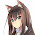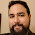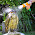Saturday, August 3, 2013

Composable streaming folds

The Haskell Prelude provides multiple ways to fold lists into a single value. For example, you can count the number of elements in a list:
import Data.List (genericLength)

genericLength :: (Num i) => [a] -> i
... or you can add them up:
import Prelude hiding (sum)

-- I'm deviating from the Prelude's sum, which leaks space
sum :: (Num a) => [a] -> a
sum = foldl' (+) 0
Individually, these two folds run in constant memory when given a lazy list as an argument, never bringing more than one element into memory at a time:
>>> genericLength [1..100000000]
100000000
>>> sum' [1..100000000]
5000000050000000
However, we get an immediate space leak if we try to combine these two folds to compute an average:
>>> let average xs = sum xs / genericLength xs
>>> average [1..100000000]
<Huge space leak>
The original isolated folds streamed in constant memory because Haskell is lazy and does not compute each element of the list until the fold actually requests the element. After the fold traverses each element the garbage collector detects the element will no longer be used and collects it immediately, preventing any build-up of elements.

However, when we combine these two folds naively like we did with average then our program leaks space while we compute sum and before we get a chance to compute genericLength. As sum traverses the list, the garbage collector cannot collect any of the elements because we have to hold on to the entire list for the subsequent genericLength fold.

Unfortunately, the conventional solution to this is not pretty:
mean :: [Double] -> Double
mean = go 0 0
where
go s l []     = s / fromIntegral l
go s l (x:xs) = s `seq` l `seq`
go (s+x) (l+1) xs
Here we've sliced open the guts of each fold and combined their individual step functions into a new step function so we can pass over the list just once. We also had to pay a lot of attention to detail regarding strictness. This is what newcomers to Haskell complain about when they say you need to be an expert at Haskell to produce highly efficient code.

The Fold type

Let's fix this by reformulating our original folds to preserve more information so that we can transparently combine multiple folds into a single pass over the list:
{-# LANGUAGE ExistentialQuantification #-}

import Data.List (foldl')
import Data.Monoid

data Fold a b = forall w.  (Monoid w) => Fold
{ tally     :: a -> w
, summarize :: w -> b
}

fold :: Fold a b -> [a] -> b
fold (Fold t c) xs =
c (foldl' mappend mempty (map t xs))
Here I've taken a fold and split it into two parts:
• tally: The step function that we use to accumulate each element of the list
• summarize: The final function we call at the end of the fold to convert our accumulator into the desired result
The w type variable represents the internal accumulator that our Fold will use as it traverses the list. The Fold can use any accumulator of its choice as long as the accumulator is a Monoid of some sort. We specify that in the types by existentially quantifying the accumulator using the ExistentialQuantification extension.

The end user also doesn't care what the internal accumulator is either, because the user only interacts with Folds using the fold function. The type system enforces that fold (or any other function) cannot use any specific details about a Fold's accumulator other than the fact that the accumulator is a Monoid.

We'll test out this type by rewriting out our original folds using the new Fold type:
genericLength :: (Num i) => Fold a i
genericLength =
Fold (\_ -> Sum 1) (fromIntegral . getSum)

sum :: (Num a) => Fold a a
sum = Fold Sum getSum
Notice how the Monoid we choose implicitly encodes how to accumulate the result. genericLength counts the number of elements simply by mapping them all to Sum 1, and then the Monoid instance for Sum just adds up all these ones to get the list length. sum is even simpler: just wrap each element in Sum and the Monoid instance for Sum adds up every element of the list. When we're done, we unwrap the final result using getSum.

We can now apply these folds to any list using the fold function, which handles all the details of accumulating each element of the list and summarizing the result:
>>> fold genericLength [(1::Int)..100000000]
100000000
>>> fold sum [(1::Int)..100000000]
5000000050000000
So far, so good, but how do we combine them into an average?

Combining Folds

Fold has the nice property that it is an Applicative, given by the following definition:
import Control.Applicative
import Data.Strict.Tuple

instance (Monoid a, Monoid b) => Monoid (Pair a b) where
mempty = (mempty :!: mempty)
mappend (aL :!: aR) (bL :!: bR) =
(mappend aL bL :!: mappend aR bR)

instance Functor (Fold a) where
fmap f (Fold t k) = Fold t (f . k)

instance Applicative (Fold a) where
pure a    = Fold (\_ -> ()) (\_ -> a)
(Fold tL cL) <*> (Fold tR cR) =
let t x = (tL x :!: tR x)
c (wL :!: wR) = (cL wL) (cR wR)
in  Fold t c
Note that this uses strict Pairs from Data.Strict.Tuple to ensure that the combined Fold still automatically runs in constant space. You only need to remember that (x :!: y) is the strict analog of (x, y).

With this Applicative instance in hand, we can very easily combine our sum and genericLength folds into an average fold:
average :: (Fractional a) => Fold a a
average = (/) <\$> sum <*> genericLength
This combines the two folds transparently into a single fold that traverses the list just once in constant memory, computing the average of all elements within the list:
>>> fold average [1..1000000]
500000.5
Now we're programming at a high-altitude instead of hand-writing our own accumulators and left folds and praying to the strictness gods.

What if we wanted to compute the standard deviation of a list? All we need is one extra primitive fold that computes the sum of squares:
sumSq :: (Num a) => Fold a a
sumSq = Fold (\x -> Sum (x ^ 2)) getSum
Now we can write a derived fold using Applicative style:
std :: (Floating a) => Fold a a
std =  (\ss s len -> sqrt (ss / len - (s / len)^2))
<\$> sumSq
<*> sum
<*> genericLength
... which still traverses the list just once:
fold std [1..10000000]
2886751.345954732
In fact, this is the exact same principle that the BIRCH data clustering algorithm uses for clustering features. You keep a tally of the length, sum, and sum of squares, and you can compute most useful statistics in O(1) time from those three tallies.

Similarly, what if we wanted to compute both the sum and product of a list in a single pass?
product :: (Num a) => Fold a a
product = Fold Product getProduct
Once again, we can just use Applicative style:
>>> fold ((,) <\$> sum <*> product) [1..100]
(5050,9332621544394415268169923885626670049071596826438162146859
2963895217599993229915608941463976156518286253697920827223758251
185210916864000000000000000000000000)

Conclusion

Contrary to conventional wisdom, you can program in Haskell at a high level without leaking space. Haskell gives you the tools to abstract away efficient idioms behind a convenient and composable interface, so use them!

Appendix

I've included the full code so that people can play with this themselves:
{-# LANGUAGE ExistentialQuantification #-}

import Control.Applicative
import Data.List (foldl')
import Data.Monoid
import Data.Strict.Tuple
import Prelude hiding (sum, length)

data Fold a b = forall w.  (Monoid w) => Fold
{ tally   :: a -> w
, compute :: w -> b
}

fold :: Fold a b -> [a] -> b
fold (Fold t c) xs =
c (foldl' mappend mempty (map t xs))

instance (Monoid a, Monoid b) => Monoid (Pair a b) where
mempty = (mempty :!: mempty)
mappend (aL :!: aR) (bL :!: bR) =
(mappend aL bL :!: mappend aR bR)

instance Functor (Fold a) where
fmap f (Fold t k) = Fold t (f . k)

instance Applicative (Fold a) where
pure a    = Fold (\_ -> ()) (\_ -> a)
(Fold tL cL) <*> (Fold tR cR) =
let t x = (tL x :!: tR x)
c (wL :!: wR) = (cL wL) (cR wR)
in  Fold t c

genericLength :: (Num b) => Fold a b
genericLength =
Fold (\_ -> Sum (1::Int)) (fromIntegral . getSum)

sum :: (Num a) => Fold a a
sum = Fold Sum getSum

sumSq :: (Num a) => Fold a a
sumSq = Fold (\x -> Sum (x ^ 2)) getSum

average :: (Fractional a) => Fold a a
average = (\s c -> s / c) <\$> sum <*> genericLength

product :: (Num a) => Fold a a
product = Fold Product getProduct

std :: (Floating a) => Fold a a
std =  (\ss s len -> sqrt (ss / len - (s / len)^2))
<\$> sumSq
<*> sum
<*> genericLength

1.Great article!

Shouldn't the genericLength use Integer instead of Int in the Sum monoid to avoid possible overflow, though?

1.Yes, it should. I used `Int` for efficiency but then forgot that this completely defeats the purpose of `genericLength`. I will fix it.

2.Shouldn't a more generic version use whatever the result type is rather than Integer, which is horrifically slow? (Say were I working with Word64.)

3.Yeah, you are right. That makes much more sense. I will fix this.

2.`fold f xs = case f of
Fold t c -> c (foldl' mappend mempty (map t xs))`

why not
`fold (Fold t c) xs = c (foldl' mappend mempty (map t xs))`

1.For some weird reason I thought that you needed a case statement to unpack an existentially quantified data type. Even weirder, I somehow got it right on the `Functor` instance without even realizing what I was doing.

Thanks for pointing this out! I fixed that and the Applicative instance as well.

3.`average = (/) <\$> sum <*> length`

should it be

`average = (/) <\$> sum <*> genericLength`

?

1.Yeah, you're right. I fixed it.

4.In my formulation of this technique (http://squing.blogspot.com/2008/11/beautiful-folding.html), there is no Monoid constraint on the accumulator technique. Does the constraint give you any extra power?

1.The monoid constraint facilitates composition, and adds convenience (mempty is used implicitly rather than providing the start value explicitly). You could accomplish the same compositional capabilities without Monoid.

2.I arrived at this from simplifying some `pipes` folding code that used `WriterT` in the base monad, so the original code had to use the `Monoid`-based approach. It's just a legacy of how I originally arrived at the problem.

I will probably write this up into a library soon and I will benchmark both approaches, too, and use the one that produces more efficient code.

3.I forgot to answer the second half of your question: the constraint does not give you extra power. See this post by Brent Yorgey which explains how foldMap and foldr are equivalent:

4.So I just tested it and your version produces strikingly more efficient code. It also solves a problem that the `Monoid`-based version does not, which is that you encode strict left fold using your version, whereas the `Monoid`-based approach cannot (while still running in constant space).

So I'm going to switch to your way.

5.Great article! I'm appreciating Applicative more and more each day.

6.Have you looked at the paper "The essence of iteration", Gabriel?
=> www.cs.ox.ac.uk/jeremy.gibbons/publications/iterator.pdf

I think the authors have the same objective. For me, this paper was very enlightening as to the understanding of the interests of Applicatives.

1.I skimmed it before but I read it more closely this time. I see what you mean now. My proposal is a special case of their using `Const` to fold and `Prod` to combine multiple folds (although it would need to be a strict `Prod`).

The main advantage to specializing it just to folds is that you get the nice `Applicative` instance for `Fold`, which you don't get if you use `Const` and `Prod` unless you formulated some sort of higher-order `Applicative`.

There is also a performance advantage if you stick to strict left folds. I've been writing this up here:

... and if you use the "free left fold" approach you get much better core, and it gets even better once you start adding rewrite rules to fuse away the intermediate list.

7.The situation with Data.List.genericLength is much messier than you describe. In fact, if you add one or two zeros to your first example, ghci will run out of stack space, but code compiled with -O will not. This is because genericLength is lazy by default (essentially written as a right fold), but has special rewrite rules for Integer and Int to make it (essentially) a strict left fold. So in optimized code, the defaulting to Integer makes that work okay, but as soon as you divide by *anything*, even a fixed constant like 2, the default turns to Double, which doesn't have that exception, and memory usage goes through the roof.

1.Huh, I never realized that. Is there any mailing list thread documenting why they chose to implement it that way?

2.I don't know what discussion went into it, but since that decision was made, Edward Kmett at least has used it for lazy Nats. The rewrite rules were an attempt to imrove efficiency in some cases, bu in my opinion were poorly conceived.

8.This might be relevant: https://hackage.haskell.org/package/folds

1.Yeah, I also created a package for the left-fold variation of this trick: http://hackage.haskell.org/package/foldl